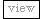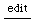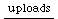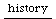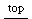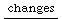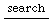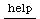## Finite State Machines

a general definition of the term: finite state machine

### Markov Chains:

also see: "artificial life"

Markov-chains are a mathematic concept of kind of series,
created from a finite (or infinite countable) number of elements.
Each of those elements has range of possible next states
that can follow this element.

So Markov Chains every element in the series depends on the
previous element only.
Markov Chains of this type are called first order markov chains, the nth order
meaning that each element is dependent on n preceding elements.Contrary to other finite state machines, like the ones used in compilers, they create a random pattern which can show unexpected behaviour.
This is maybe why they have been used a lot in algorithimic composition and there are many ways to use them.
More sophisticated information

In SuperCollider there is an implementation to be found as a subclass of ListPattern
in which one can use Patterns as elements and Arrays of indices as possible next states.
The structure looks like this:
```	Pfsm([
[ entry indices ],
pattern, [ indices ],
pattern, [ indices ],
pattern, [ indices ],
pattern, [ indices ],
:
:
:
nil, nil //terminal states
])
```

a good example is to be found at http://www.audiosynth.com/schtmldocs/Examples/Patterns-Markov.html

the trick with this simple layout is that next index is just picked from the array of indices ("followups").
The more there are of one index, the highter is the probability of getting chosen.

this example shows a way to make a markov chain maker:

```
//a Pfsm maker (sc2/3)
(
var fsmaker;
fsmaker = { arg pat, n, rando;
var machine;
machine = Array.fill(n, { arg i;
[pat, Array.fill(rando, { n.rand })]
}).flatten(1);
machine = [] ++ machine ++ [nil, nil];
Pfsm(machine);
};
a = Pbind(
\degree, fsmaker.value(Pshuf([1, 2, -3, 14, 5, 16], 2), 4, 5),
\dur, fsmaker.value(Pshuf([0.1, 0.2, 0.3], 4)*0.6, 3, 5));
a = fsmaker.value(a, 15, 8).play;
)

//producing Pfsm specs ((sc2/3)
(
var n, patterns, probabilities, specs;
n = 4;
patterns = Array.fill(n, { Pseq(Array.fill(rrand(2, 6),{ 1.0.rand }), [1, 2, 3].choose) });
probabilities = Array.fill(n+1, { Array.fill(5, {(n-1).rand }) });
specs = [probabilities.at(0)];

(probabilities.size-1).do({ arg i; specs = specs.add(patterns.at(i));  specs = specs.add(probabilities.at(i+1)) });
p = Pfsm(specs ++ [nil, nil]);

Pseq([
Pbind(
\dur, 0.1,
\amp, p,
\legato, 0.5,
// \freq, p*200+250
\degree, p*12
)
], inf).play;
)
```

...to be continued
please feel free to contribute
authors so far:
jrh
...